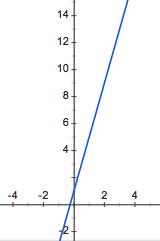### Home > APCALC > Chapter 1 > Lesson 1.3.3 > Problem1-131

1-131.

Examine two ways a line changes:

1. Sketch $f(x) = 4x + 1$. What are $f(0), f(1), f(2), \text{ and }f(3)$? How are the function values changing as $x$ increases?Compute the finite differences (that is, find the difference in $y$-values as $x$ increases) and look for a pattern.

2. Calculate the area under the curve for $0 ≤ x ≤ a$ if $a = 0, 1, 2, \text{ and } 3$. How is the area changing as $a$ increases?

Use geometry to find the area of the trapezoid between $x = 0$ and $x = 2$ for each value of $a$. Keep your results organized.

Is the difference between the areas growing constantly or linearly? If not, how else could it be growing, and by what pattern each time?